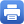Columbia Home
2013 Kolchin Lecture with Prof. Burt Totaro

The spring 2013 iteration of the Ellis R. Kolchin Memorial Lecture will be delivered by Prof. Burt Totaro (UCLA)on Monday, April 15th, 2013. Prof. Totaro will give the following lecture:

The Hodge Conjecture: History and Recent Progress

Abstract: A basic issue in algebraic geometry is the relation between continuous functions (topology) and polynomial functions over the complex numbers (algebraic geometry). The Hodge conjecture is a major problem about this relation: roughly, when can an even-dimensional real submanifold of a complex algebraic manifold be moved continuously to a complex algebraic submanifold? We discuss the history and some recent advances, notably on the integral Hodge conjecture in dimension 3.

Monday, April 15, 2013 at 4:15pm
Room 207 MathematicsPrint this page